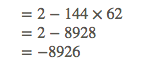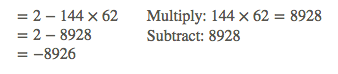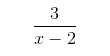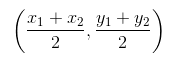# Math Editor: LaTex Equation Reference

This article lists a few sample LaTex equations for use in our math editor.

Multi-line equations

• To align, you should use the Blockmath option, by clicking the appropriate radio button below the text entry window in the equation editor.
• The location of the & symbol indicates the point of horizontal alignment.
• Lines must be ended with \\.
• The ‘\’, ‘\quad\’, and ‘\qquad’ commands force 1, 4, and 8 blank spaces, respectively.

Example 1:Latex Code:

\begin{align}
&= 2-144 \times 62 && \text{Multiply:} \ 144 \times 62 = 8928\\
&= 2 - 8928 && \text{Subtract: 8928}\\
&= - 8926
\end{align}

Example 2:Latex Code:

\begin{align}
&= 2-144 \times 62 \\
&= 2 - 8928 \\
&= - 8926
\end{align}

Fractions

Example 1:Latex Code:

\frac{3}{x-2}

Example 2:Latex Code:

\left( \frac{x_1+x_2}{2}, \frac{y_1+y_2}{2} \right)